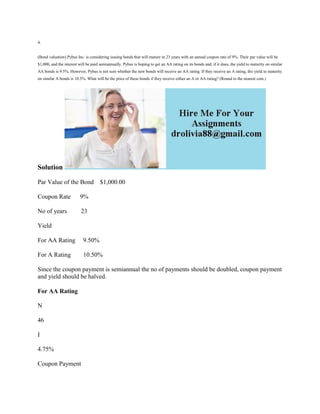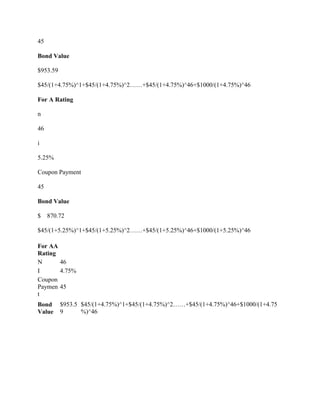Ce diaporama a bien été signalé.
Le téléchargement de votre SlideShare est en cours. ×

# 4- (Bond valuation) Pybus Inc- is considering issuing bonds that will.docx

Publicité
Publicité
Publicité
Publicité
Publicité
Publicité
Publicité
Publicité
Publicité
Publicité
PublicitéChargement dans…3
×

## Consultez-les par la suite

1 sur 3 Publicité

# 4- (Bond valuation) Pybus Inc- is considering issuing bonds that will.docx

4.
(Bond valuation) Pybus Inc. is considering issuing bonds that will mature in 23 years with an annual coupon rate of 9%. Their par value will be \$1,000, and the interest will be paid semiannually. Pybus is hoping to get an AA rating on its bonds and, if it does, the yield to maturity on similar AA bonds is 9.5%. However, Pybus is not sure whether the new bonds will receive an AA rating. If they receive an A rating, the yield to maturity on similar A bonds is 10.5%. What will be the price of these bonds if they receive either an A or AA rating? (Round to the nearest cent.)
Solution
Par Value of the Bond    \$1,000.00
Coupon Rate      9%
No of years         23
Yield
For AA Rating     9.50%
For A Rating        10.50%
Since the coupon payment is semiannual the no of payments should be doubled, coupon payment and yield should be halved.
For AA Rating
N
46
I
4.75%
Coupon Payment
45
Bond Value
\$953.59
\$45/(1+4.75%)^1+\$45/(1+4.75%)^2……+\$45/(1+4.75%)^46+\$1000/(1+4.75%)^46
For A Rating
n
46
i
5.25%
Coupon Payment
45
Bond Value
\$    870.72
\$45/(1+5.25%)^1+\$45/(1+5.25%)^2……+\$45/(1+5.25%)^46+\$1000/(1+5.25%)^46

.

4.
(Bond valuation) Pybus Inc. is considering issuing bonds that will mature in 23 years with an annual coupon rate of 9%. Their par value will be \$1,000, and the interest will be paid semiannually. Pybus is hoping to get an AA rating on its bonds and, if it does, the yield to maturity on similar AA bonds is 9.5%. However, Pybus is not sure whether the new bonds will receive an AA rating. If they receive an A rating, the yield to maturity on similar A bonds is 10.5%. What will be the price of these bonds if they receive either an A or AA rating? (Round to the nearest cent.)
Solution
Par Value of the Bond    \$1,000.00
Coupon Rate      9%
No of years         23
Yield
For AA Rating     9.50%
For A Rating        10.50%
Since the coupon payment is semiannual the no of payments should be doubled, coupon payment and yield should be halved.
For AA Rating
N
46
I
4.75%
Coupon Payment
45
Bond Value
\$953.59
\$45/(1+4.75%)^1+\$45/(1+4.75%)^2……+\$45/(1+4.75%)^46+\$1000/(1+4.75%)^46
For A Rating
n
46
i
5.25%
Coupon Payment
45
Bond Value
\$    870.72
\$45/(1+5.25%)^1+\$45/(1+5.25%)^2……+\$45/(1+5.25%)^46+\$1000/(1+5.25%)^46

.

Publicité
Publicité

### 4- (Bond valuation) Pybus Inc- is considering issuing bonds that will.docx

1. 1. 4. (Bond valuation) Pybus Inc. is considering issuing bonds that will mature in 23 years with an annual coupon rate of 9%. Their par value will be \$1,000, and the interest will be paid semiannually. Pybus is hoping to get an AA rating on its bonds and, if it does, the yield to maturity on similar AA bonds is 9.5%. However, Pybus is not sure whether the new bonds will receive an AA rating. If they receive an A rating, the yield to maturity on similar A bonds is 10.5%. What will be the price of these bonds if they receive either an A or AA rating? (Round to the nearest cent.) Solution Par Value of the Bond \$1,000.00 Coupon Rate 9% No of years 23 Yield For AA Rating 9.50% For A Rating 10.50% Since the coupon payment is semiannual the no of payments should be doubled, coupon payment and yield should be halved. For AA Rating N 46 I 4.75% Coupon Payment
2. 2. 45 Bond Value \$953.59 \$45/(1+4.75%)^1+\$45/(1+4.75%)^2……+\$45/(1+4.75%)^46+\$1000/(1+4.75%)^46 For A Rating n 46 i 5.25% Coupon Payment 45 Bond Value \$ 870.72 \$45/(1+5.25%)^1+\$45/(1+5.25%)^2……+\$45/(1+5.25%)^46+\$1000/(1+5.25%)^46 For AA Rating N 46 I 4.75% Coupon Paymen t 45 Bond Value \$953.5 9 \$45/(1+4.75%)^1+\$45/(1+4.75%)^2……+\$45/(1+4.75%)^46+\$1000/(1+4.75 %)^46